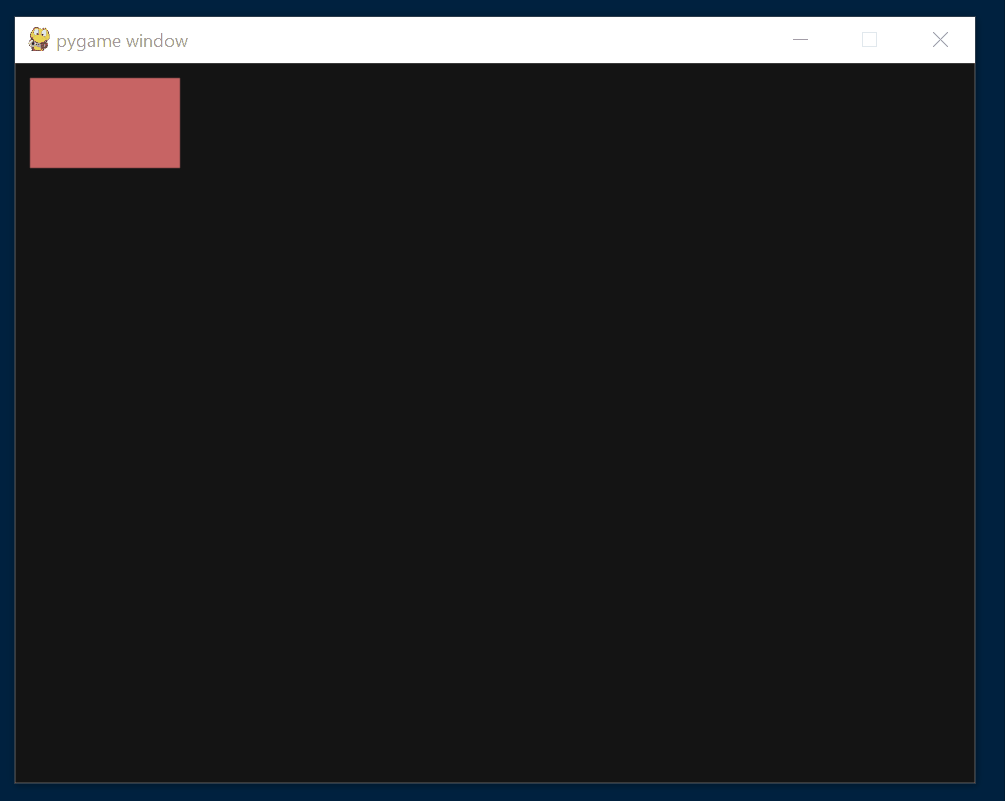# The collidepoint() Method in Pygame

Maxim Maeder May 11, 2022

This article shows how to draw text in Python using Pygame. This is not the complete code for a working Python game window.

If you are interested in a bare-bones working Framework, check this article.

## Use the `collidepoint()` Method in Pygame

In the `collidepoint()` method of the `rect` class, we test if a given point is inside the `rect`. Next, we will make a button that changes color when hovering over it.

You can either supply the function with the x and y coordinate separately or provide them in an iterable, like a tuple or list.

Syntax:

``````rect.collidepoint(x, y)
rect.collidepoint((x, y))
``````

Now we use `collidepoint()` to change the color of a surface when we hover over it. We do the following things to set this up.

1. Start by defining a `rect` using the `collidepoint()` method.
2. Continue by making a surface with the specified `rect` width and height.
3. The last thing we do before the main loop will be to make two variables. One represents the normal color and one the hover color.
``````# Before Main loop
rect = pygame.Rect(10, 10, 100, 60)
btn_surface = pygame.Surface(
(rect.width, rect.height)
)

normal_color = (200, 100, 100)
hover_color = (100, 200, 100)
``````

We get the mouse position in the main loop and check if it is inside the `rect`.

1. We get the mouse position and pass it to the `collidepoint()` method of the `rect` we made above.
2. If the mouse turns out to be on top, we fill the button surface with our hover color. In all other cases, we fill it with the normal color.
3. Lastly, we blit the surface onto the screen.
``````# In the Main loop
if rect.collidepoint(pygame.mouse.get_pos()):
btn_surface.fill(hover_color)
else:
btn_surface.fill(normal_color)

screen.blit(btn_surface, rect)
``````

Complete Code:

``````# Imports
import sys
import pygame

# Configuration
pygame.init()
fps = 60
fpsClock = pygame.time.Clock()
width, height = 640, 480
screen = pygame.display.set_mode((width, height))

# Before Main loop
rect = pygame.Rect(10, 10, 100, 60)
print(rect.width)
btn_surface = pygame.Surface(
(rect.width, rect.height)
)

normal_color = (200, 100, 100)
hover_color = (100, 200, 100)

# Game loop.
while  True:
screen.fill((20, 20, 20))
for event in pygame.event.get():
if event.type == pygame.QUIT:
pygame.quit()
sys.exit()

# In the Main loop
if rect.collidepoint(pygame.mouse.get_pos()):
btn_surface.fill(hover_color)
else:
btn_surface.fill(normal_color)

screen.blit(btn_surface, rect)

pygame.display.flip()
fpsClock.tick(fps)
``````

Output:Author: Maxim Maeder

Hi, my name is Maxim Maeder, I am a young programming enthusiast looking to have fun coding and teaching you some things about programming.

GitHub